# How to plot continuous probability distributions

## Description

There are many famous continuous probability distributions, such as the normal and exponential distributions. How can we get access to them in software, to plot the distribution as a curve?

## Solution, in Excel

View this solution alone.

We begin by creating the values that will be shown on the $x$-axis. These values depend not only on the distribution, but on which portion of it you wish to see.

For example, for an exponential distribution, the sample space is $(0,\infty)$, but for a standard normal distribution, it is the whole real line, and you may wish to view just $( - 5,5)$, or some other range.

In the example below, we will use a Gamma distribution with $\alpha = 5$ and $\beta = 5$, plotted on the range $\lbrack 0,50\rbrack$, but the particular example doesn’t matter; you can use the procedure below for any distribution.

To generate the $x$ values from 0 to 50, begin with just the first two values in the sequence, in this case 0 and 1, as shown below.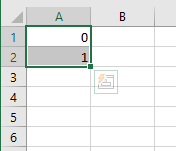Drag the small green square in the bottom right of the selection downward, to create a sequence that goes all the way up to 50. (Only the beginning of it is shown here.)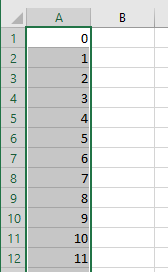If your sample spaces were a smaller range (say, just from -2 to 2), you would need to use smaller steps to get a smooth plot. For example, you might begin with -2 and -1.9 to tell Excel to take steps of size 0.1.

In the adjacent column, we put the formula for the distribution, based on the $x$ values in the first column. In this example, recall that we’ll plot a Gamma distribution with $\alpha = 5$ and $\beta = 5$, so we use the formula shown below. The final parameter for the distribution should always be FALSE, to indicate that we are not asking Excel for a cumulative distribution function, but just the usual probability density function.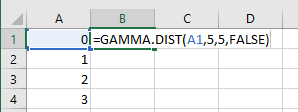After typing your probability density function’s formula, drag it down the column.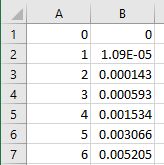Highlight just column B and insert a line chart from the Insert tab on the Ribbon, as shown below.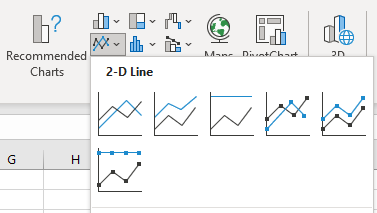This will create a chart that does not yet include your desired $x$-axis labels; rather, the horizontal axis markings will be 1, 2, 3, 4, etc. To get the correct labels on the $x$-axis, right-click the chart and choose “Select Data…” This will bring up the window shown below.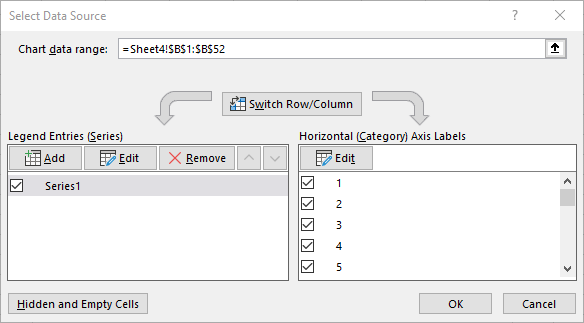Click the Edit button for the Horizontal (Category) Axis Labels and select column A. Click OK twice to return to your plot, which should then have the correct $x$-axis labels. You can then update the chart title and axis labels to be more descriptive if desired, as shown in the final result, below.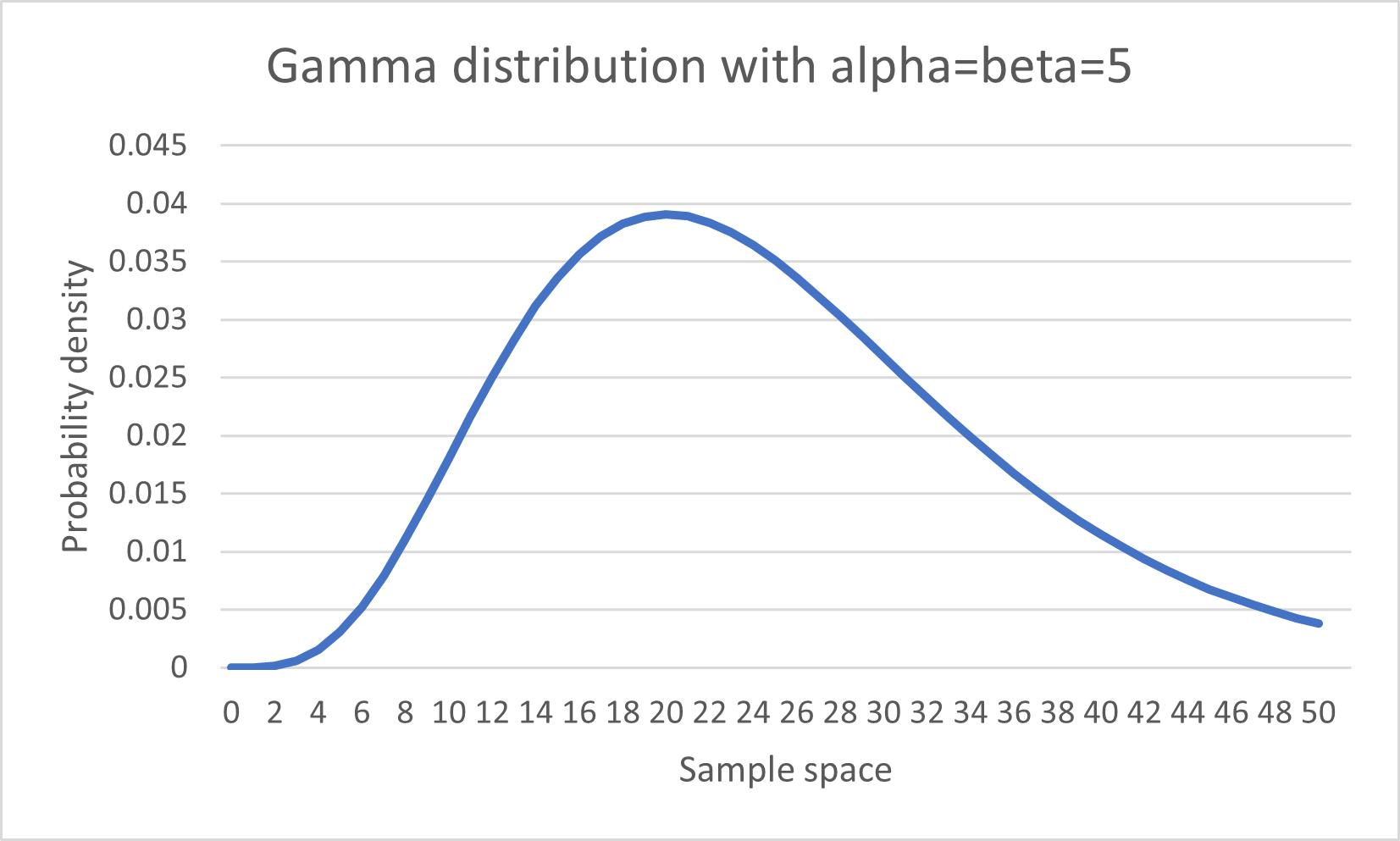Although we used the GAMMA.DIST function in Excel, you can use any of the built-in continuous probability distribution functions, such as BETA.DIST, CHISQ.DIST, F.DIST, NORM.DIST, LOGNORM.DIST, or T.DIST.

See a problem? Tell us or edit the source.

## Solution, in Julia

View this solution alone.

You can import many different random variables from Julia’s Distributions package. The full list of them is online here.

If you don’t have that package installed, first run using Pkg and then Pkg.add( "Distributions" ) from within Julia.

The challenge with plotting a random variable is knowing the appropriate sample space, because some random variables have sample spaces of infinite width, which cannot be plotted.

But we can just ask Julia to show us the central 99.98% of a continuous distribution, which is almost always indistinguishable to the human eye from the entire distribution.

We style the plot below so that it is clear the sample space is continuous.

1
2
3
4
5
6
7
8
9
using Distributions
X = Normal( 10, 5 )                   # use a normal distribution with μ=10 and σ=5

xmin = quantile( X, 0.0001 )          # compute min x as the 0.0001 quantile
xmax = quantile( X, 0.9999 )          # compute max x as the 0.9999 quantile
xs = range( xmin, xmax, length=100 )  # create 100 x values in that range

using Plots
plot( xs, pdf.( X, xs ) )             # plot the shape of the distribution


See a problem? Tell us or edit the source.

## Using SciPy, in Python

View this solution alone.

You can import many different random variables from SciPy’s stats module. The full list of them is online here.

The challenge with plotting a random variable is knowing the appropriate sample space, because some random variables have sample spaces of infinite width, which cannot be plotted.

But we can just ask SciPy to show us the central 99.98% of a continuous distribution, which is almost always indistinguishable to the human eye from the entire distribution.

We style the plot below so that it is clear the sample space is continuous.

1
2
3
4
5
6
7
8
9
10
11
from scipy import stats
X = stats.norm( 10, 5 )      # use a normal distribution with μ=10 and σ=5

xmin = X.ppf( 0.0001 )       # compute min x as the 0.0001 quantile
xmax = X.ppf( 0.9999 )       # compute max x as the 0.9999 quantile
import numpy as np
xs = np.linspace( xmin, xmax, 100 )  # create 100 x values in that range

import matplotlib.pyplot as plt
plt.plot( xs, X.pdf( xs ) )  # plot the shape of the distribution
plt.show()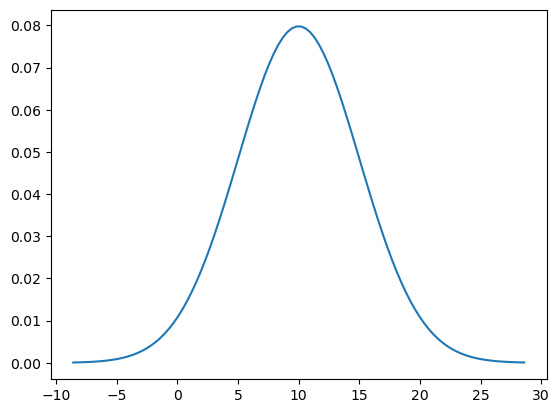See a problem? Tell us or edit the source.

## Solution, in R

View this solution alone.

Because R is designed for use in statistics, it comes with many probability distributions built in. A list of them is online here.

The challenge with plotting a random variable is knowing the appropriate sample space, because some random variables have sample spaces of infinite width, which cannot be plotted.

But we can just ask R to show us the central 99.98% of a continuous distribution, which is almost always indistinguishable to the human eye from the entire distribution.

We will use a normal distribution with $\mu=10$ and $\sigma=5$, but if you wanted to use a different distribution, you could replace qnorm and dnorm with, for example, qchisq and dchisq (for the $\chi^2$ distribution), adjusting the named parameters as appropriate. (For a list of supported distributions, see the link above.)

We style the plot below so that it is clear the sample space is continuous.

1
2
3
4
5
xmin <- qnorm( 0.0001, mean=10, sd=5 )  # compute min x as the 0.0001 quantile
xmax <- qnorm( 0.9999, mean=10, sd=5 )  # compute max x as the 0.9999 quantile
xs <- seq( xmin, xmax, length.out=100 ) # create 100 values in that range
ys <- dnorm( xs, mean=10, sd=5 )        # compute the shape of the distribution
plot( xs, ys, type='l' )                # plot that shape as a smooth line